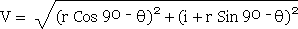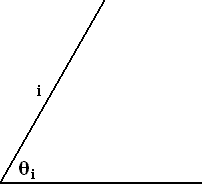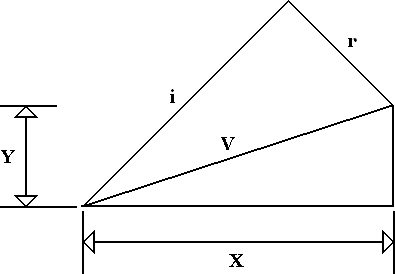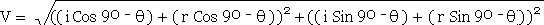# Antenna Voltage, Phase, Power and Impedance Distribution page 3

The voltage at any point along the antenna in the INCIDENT direction can be calculated from the input voltage, the distance of the point from the input in terms of phase shift (θi) and the voltage drop coefficient using :-

V = Vin - ( Cv x θi)   Equation (2.1)

Where Vin is the input voltage in Volts

Cv is the coefficient for voltage drop /°

θi is the distance from the input of the antenna in degrees

For the voltage at any point along the antenna in the REFLECTED direction the formula becomes :-

V = Vin - (Cv x (90 + θe))   Equation (2.2)

θe is the distance from the end of the antenna in degrees

The vector sum is calculated at each point using the INCIDENT voltage at 90° (which is the peak INCIDENT voltage at that point) and the phase angle (θ) as the difference between the INCIDENT and REFLECTED phases, and these vectors are shown in figure 6.

Figure 6 Vectors to be AddedAddition method as illustrated in figure 7.

Figure 7 Vector SumFirst calculate the magnitude of the X component with i = 0 :-

X = r Cos (90 - θ)

Calculate the Y component :-

Y = i + r Sin (90 - θ)

Calculate the Vector Sum from X and Y :-

V = (X2 + Y2)0.5Equation (2.3)

Equation 2.3 is used to calculate the Vector Sum at each 10° interval and a graph is made as in figure 8. The calculated theoretical INCIDENT and REFLECTED voltage using equations 2.1 and 2.2 have been included in the graph in order to give a comparative picture.

Figure 8It can be seen from figure 8 that when a linear phase shift is assumed, a curve generated from INCIDENT and REFLECTED voltages into a vector sum voltages using the principles of a linear voltage drop that does not reach zero fits well with an actual measured sum voltage curve. The top two measured points being a little high because of the probe being affected by direct radiation through the plastic end, where there is no screening.

It has now also been shown that the indicated voltage (1.02 V) at the end of the antenna is less than twice the calculated input voltage (0.6 V) and is not a 'theoretical' infinite voltage! It is possible to have high voltages generated when an 'electrically short' antenna is matched to a transmission line with an inductor because this is a magnification network. In which case the antenna acts as a capacitor and combined with the inductor forms a resonant circuit which magnifies the voltages present. That is a different mechanism to the apparent 'voltage doubling' that is observed here with an antenna that is well matched to the feeder.

Now that the voltage distribution is established and it can be seen that the voltage drops along the length of the antenna in a linear manner the phase shift can be measured and calculated. The phase is measured in the same manner as the voltage measurement, with the VNA gating on and the probe moved at intervals along the length of the antenna. The results have to be incremented by approximately 90° because the VNA phase measurements are referenced to the calibration point at the input of the antenna whereas mathematically the phase is generally more easily referenced with 0° at the end of the antenna. Thus giving positive phase in the INCIDENT direction and negative phase in the REFLECTED direction. The simplest solution being to add approximately 90° to the phase measurement results at the beginning of measurement analysis. The raw results are not linear and once again we have a vector sum result and the data has to be processed in order to extract the INCIDENT and REFLECTED phases.

Figure 9The vector voltages are taken at their respective phase angles as per figure 10 with the REFLECTED direction giving a negative voltage.

Figure 10 INCIDENT and REFLECTED Vectors to be AddedThe X and Y coordinates are calculated before being resolved into a vector sum using the Triangle method as illustrated in figure 11.

Figure 11 Vector SumFirst calculate the magnitude of the X component with i = 0 :-

X = i Cos (90 - θ) + r Cos (90 - θ)

Calculate the Y component :-

Y = i Sin (90 - θ) + r Sin (90 - θ)

Calculate the Vector Sum from X and Y :-

V = (X2 + Y2)0.5Equation (2.4)

Equation 2.4 is used to calculate the Vector Sum at each 10° interval and and the curve generated is added to the graph as in figure 12.

Figure 12It can be seen from figure 12 that when a measured phase distribution is compared to the phase generated by the measured and processed voltages with an assumed linear phase shift, the curves are similar but the antenna phase has been pulled by the probe. However, this is not a conclusive proof of phase linearity because it is a 'circular calculation' and we shall return to this matter later in paragraph 6.2.

1  2  3  4   >  Pages

How Does an Antenna Work Index

Technical Article Index